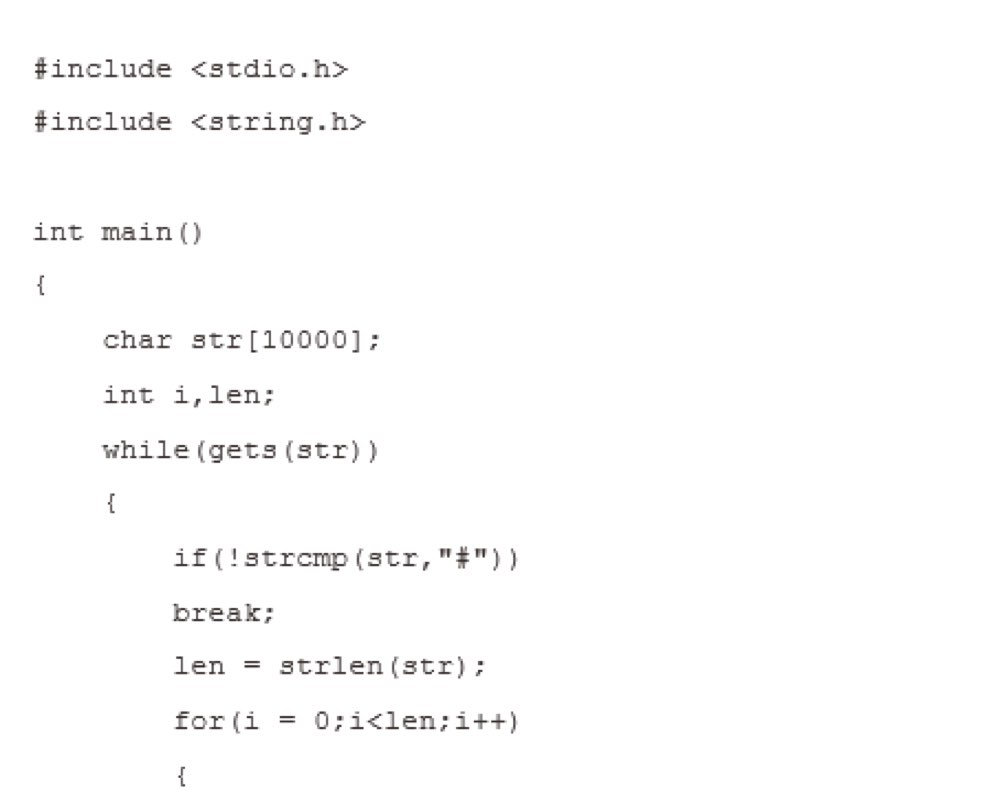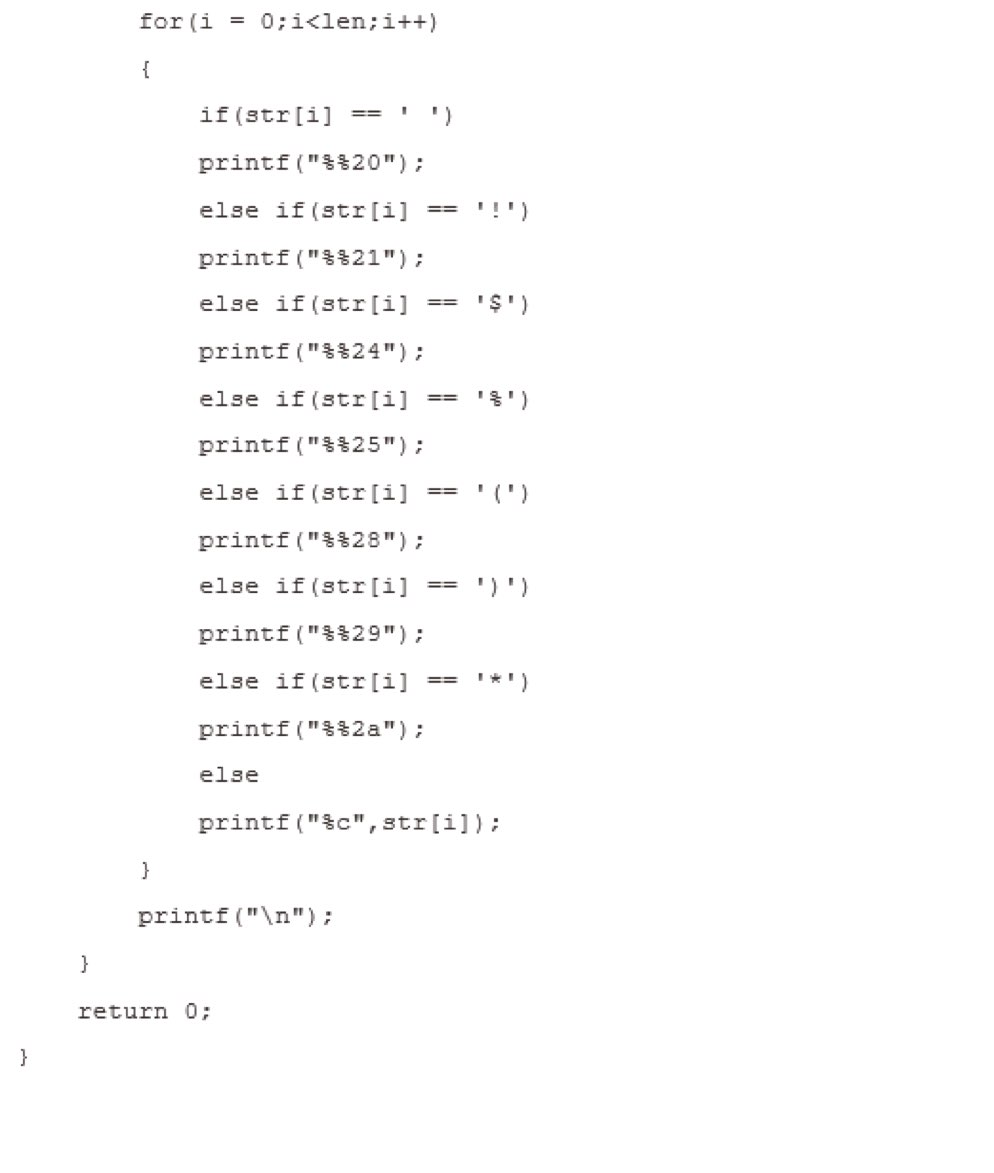2016-05-18 11:45

# 这段c语言代码换成java是什么呢？求教啊！？

⋯⋯⋯⋯⋯⋯⋯⋯⋯⋯⋯⋯⋯⋯⋯⋯⋯⋯⋯⋯⋯⋯⋯⋯⋯⋯⋯⋯⋯⋯⋯⋯⋯⋯⋯⋯⋯⋯⋯⋯⋯⋯⋯⋯⋯⋯⋯⋯⋯⋯⋯⋯⋯⋯⋯⋯⋯⋯⋯⋯⋯⋯⋯⋯⋯⋯• 写回答
• 关注问题
• 收藏
• 邀请回答

#### 3条回答默认 最新

•魏春雨 2016-05-18 12:31
已采纳

package javaOJ;

import java.util.Scanner;

public class c {
public static void main(String[] args) {
String s;
//char[] s = new char;
Scanner scanf = new Scanner(System.in);
while(true)
{
s = scanf.next();
char[] str = s.toCharArray();
// System.out.println(cs);
if(str=='#') break;
// System.out.println(cs.length);
int len=str.length;
for(int i=0;i<len;i++)
{
if(str[i]==' ')
System.out.print("%20");
else if(str[i]=='!')
System.out.print("%21");
else if(str[i]=='\$')
System.out.print("%24");
else if(str[i]=='%')
System.out.print("%25");
else if(str[i]=='(')
System.out.print("%28");
else if(str[i]==')')
System.out.print("%29");
else if(str[i]=='*')
System.out.print("%2a");
else
System.out.print(str[i]);
}
System.out.println();
}
}
}

打赏 评论
•havedream_one 2016-05-18 23:23
``````package javaOJ;

import java.util.Scanner;

public class c {
public static void main(String[] args) {
String s;
//char[] s = new char;
Scanner scanf = new Scanner(System.in);
while(true)
{
s = scanf.next();
char[] str = s.toCharArray();
// System.out.println(cs);
if(str=='#') break;
// System.out.println(cs.length);
int len=str.length;
for(int i=0;i<len;i++)
{
if(str[i]==' ')
System.out.print("%20");
else if(str[i]=='!')
System.out.print("%21");
else if(str[i]=='\$')
System.out.print("%24");
else if(str[i]=='%')
System.out.print("%25");
else if(str[i]=='(')
System.out.print("%28");
else if(str[i]==')')
System.out.print("%29");
else if(str[i]=='*')
System.out.print("%2a");
else
System.out.print(str[i]);
}
System.out.println();
}
}
}

``````
打赏 评论
•103style 2016-05-19 05:01

意思就是替换数组中一些需要转码的字符

打赏 评论